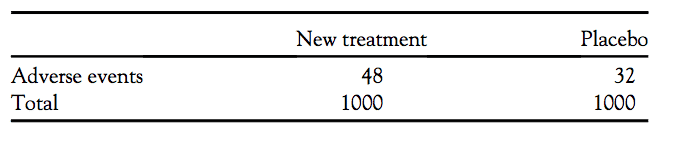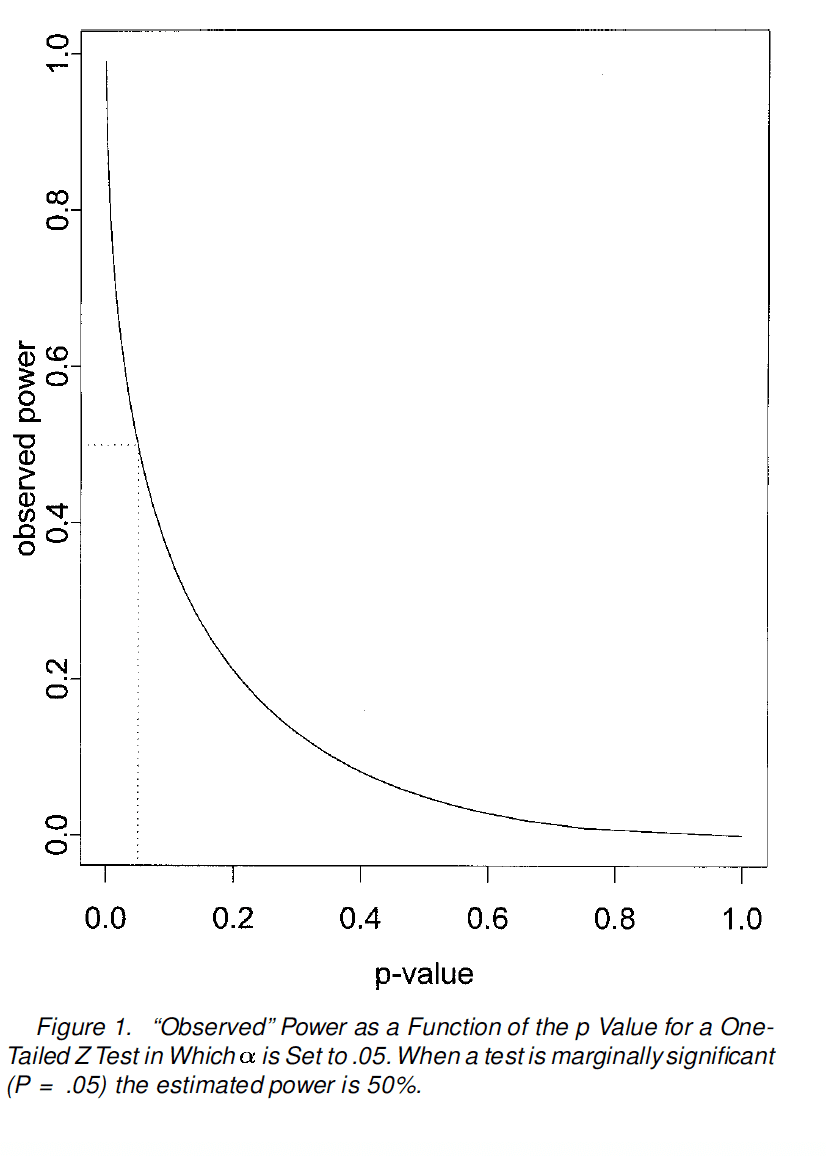# High Statistical Power Can Be Deceiving

Even though many researchers are now acquainted with what power is and why we try to aim for high power in studies, there are still several misconceptions about statistical power floating around.

For example, if a study designed for 95% power fails to find a difference between two groups, does that offer more support for the null hypothesis? Many will answer yes, because they elicit that if such a large study failed to find a difference between two groups, then this provides evidence for no effect. This sort of thinking is dangerous for several reasons that I’ll discuss in this blog post.

First, let’s use an example that Greenland (2012) provides. Let’s say we have a clinical trial testing the effects of drug X against placebo, and a primary outcome is adverse events. We have a total of 2,000 participants, and we’ve randomized 1,000 to the treatment and 1,000 to the placebo group. Here’s our contingency table (we’re working with risk ratios).Let’s say we expected to get 32 adverse events in the placebo group. If the alternative hypothesis is that the risk ratio (RR) is 2, then this design (at the 5% alpha level) gives us 85% power. So our study has a lot of statistical design power to detect a doubling of risk. If we run a significance test for the null hypothesis, which is RR = 1, then we get a p-value of 0.07. Okay, so we have high design power (85%), but we didn’t get a statistically significant difference (p=0.07).

So again, many may look at the results of this study and claim that if we have such a large sample and high design power and we didn’t find a statistically significant effect, then the following may have happened:

• there really is no difference in the population (a true equivalence).

• there is an effect, but our study was too underpowered and has high sampling error (a false equivalence) (Quertemont, 2011).

Unfortunately, it’s not possible to know what the true statistical power of our test is, so we cannot know for sure which of the scenarios above occurred.

So what can we look at to provide us with more information?

Some other basic statistical comparisons seem to give more insight than statistical power, which is no longer relevant after we have these data (Greenland, 2012; Hoenig & Heisey, 2001).

• For example, the actual point estimate risk ratio is 1.5. In ratio terms, this is closer to the alternative hypothesis (RR=2) than it is to the null (RR=1).

• The confidence interval for this point estimate (0.97, 2.33) leans towards the alternative (RR=2), while the lower bound is barely below the null (RR=1).

• The likelihood ratio comparing the alternative hypothesis (RR=2) to the null (RR=1) is 2.3.

• The p-value for the alternative hypothesis is 0.20, which is about three times the p-value for the null hypothesis (0.07).

So all of the statistical comparisons seem to support the alternative. However, a nonsignificant p-value such as 0.07 in a study that is this large and with this much design power may yield conclusions such as, “there is no difference in adverse events between the drug X group and the placebo group.”

The other argument may be that statistical power was never high and that if we calculated power using the effect size from the trial, it will clearly indicate that we never achieved high power. This too is flawed, not only because observed power (calculating power from the effect size in the study) is not the same as the actual power of our test, but also because observed power suffers from the power approach paradox.

Hoenig & Heisey (2001) gives us an excellent example to understand this phenomenon.

Let’s say that we had two experiments that result in nonsignificant results at the 5% level. The first experiment has higher observed statistical power than the second. Again, this (the result from the first experiment) may be taken as stronger evidence for the null hypothesis when compared to the second experiment, because there is more observed statistical power and the result is still nonsignificant. However, this is also incorrect.

To show why let’s look at the imaginary test statistics for the two tests. If a one-sided Z test was used for both experiments, then the Z statistic for the experiment with higher observed power (Zhp) will be larger than the Z statistic for the experiment with lower observed power (Zlp), because power is a function of Z statistic. So as power increases, the Z statistic will also increase.

And everyone knows, that larger test statistics will also yield… smaller p-values. This is also because power (observed power) is a fixed transformation of the p-value, which will shrink as power grows (Hoenig & Heasey, 2001). Thus, the first experiment (which has higher power and a larger test statistic (Zhp) will have a smaller p-value than the second experiment.So if anything, studies with higher observed power will yield results that go against the null hypothesis. If we can’t conclude that a nonsignificant result is evidence for the null in a seemingly high-powered study, then what can we conclude?

Deborah Mayo points us to a relevant passage by Jacob Cohen (the popularizer of power analysis),

Research reports in the literature are frequently flawed by conclusions that state or imply that the null hypothesis is true. For example, following the finding that the difference between two sample means is not statistically significant, instead of properly concluding from this failure to reject the null hypothesis that the data do not warrant the conclusion that the population means differ, the writer concludes, at least implicitly, that there is no difference.

The latter conclusion is always strictly invalid, and is functionally invalid as well unless power is high. The high frequency of occurrence of this invalid interpretation can be laid squarely at the doorstep of the general neglect of attention to statistical power in the training of behavioral scientists.

What is really intended by the invalid affirmation of a null hypothesis is not that the population ES is literally zero, but rather that it is negligible, or trivial. This proposition may be validly asserted under certain circumstances. Consider the following: for a given hypothesis test, one defines a numerical value i (or iota) for the ES, where i is so small that it is appropriate in the context to consider it negligible (trivial, inconsequential).

Power (1 – b) is then set at a high value, so that b is relatively small. When, additionally, a is specified, n can be found. Now, if the research is performed with this n and it results in nonsignificance, it is proper to conclude that the population ES is no more than i, i.e., that it is negligible; this conclusion can be offered as significant at the b level specified.

In much research, “no” effect (difference, correlation) functionally means one that is negligible; “proof” by statistical induction is probabilistic. Thus, in using the same logic as that with which we reject the null hypothesis with risk equal to a, the null hypothesis can be accepted in preference to that which holds that ES = i with risk equal to b.

Since i is negligible, the conclusion that the population ES is not as large as i is equivalent to concluding that there is “no” (nontrivial) effect. This comes fairly close and is functionally equivalent to affirming the null hypothesis with a controlled error rate (b), which, as noted above, is what is actually intended when null hypotheses are incorrectly affirmed (Cohen, 1988).

As indicated above, Cohen argues that when designing a study looking to detect at least an effect size of i, a nonsignificant result does not provide evidence in favor of the null hypothesis (for example, one centered on a zero effect). Rather it should lead us to conclude that the data are no more extreme than our chose effect size (i), which may be trivial. However, this is not equivalent to saying that there is absolutely no effect at all. Simply that the difference is trivial and below what we have considered being meaningful.

However, Hoenig and Heisey (2001) are not particularly convinced by Cohen’s argument,

Cohen (1988, p. 16) claimed that if you design a study to have high power 1-b to detect departure Δ from the null hypothesis, and you fail to reject the null hypothesis, then the conclusion that the true parameter value lies within Δ units of the null value is “significant at the b level. Thus, in using the same logic as that with which we reject the null hypothesis with risk equal to a, the null hypothesis can be accepted in preference to that which holds that ES [the effect size] = with risk equal to b.” (We have changed Cohen’s notation in the above to conform to that used here.)

Furthermore, Cohen stated (p. 16) “‘proof’ by statistical induction is probabilistic” without elaboration. He appeared to be making a probabilistic statement about the true value of the parameter which is invalid in a classical statistical context. Furthermore, because his procedure chooses the sample size to have a specified, fixed power before conducting the experiment, his argument assumes that the actual power is equal to the intended power and, additionally, his procedure ignores the experimental evidence about effect size and sampling variability because the value of b? is not updated according to the experimental results.

Again, Hoenig & Heisey (2001) reiterate the fact that design power does not always reflect the true power of the statistical test. They further elaborate on the first example they used to illustrate the power approach paradox,

Consider the previous two experiments where the first was closer to significance; that is, Zhp > Zlp. Furthermore, suppose that we observed the same estimated effect size in both experiments and the sample sizes were the same in both. This implies sd(hp) < sd(lp). For some desired level of power, one solves 1-φ(Z(a)√(n) *d)/sd) for d to obtain the desired detectable effect size, d.

It follows that the computed detectable effect size will be smaller in the first experiment. And, for any conjectured effect size, the computed power will always be higher in the first experiment. These results lead to the nonsensical conclusion that the first experiment provides the stronger evidence for the null hypothesis (because the apparent power is higher but significant results were not obtained), in direct contradiction to the standard interpretation of the experimental results (p values).

How can we provide better support for the null hypothesis then, if there is no difference?

A good approach is to use equivalence testing, which is discussed in depth in these papers (Lakens, 2017; Lakens, Scheel, & Isager, 2018; Meyners, 2012; Quertemont, 2011).

As Hoenig & Heisey point out,

Suppose that we are willing to conclude that a treatment is negligible if its absolute effect is no greater than some small positive value. Demonstrating such practical equivalence requires reversing the traditional burden of proof; it is not sufficient to simply fail to show a difference, one must be fairly certain that a large difference does not exist.

Thus, in contrast to the traditional casting of the null hypothesis, the null hypothesis becomes that a treatment has a large effect, or H0 : | D | ≥ Δ where D is the actual treatment effect. The alternative hypothesis is the hypothesis of practical equivalence, or HA : | D | < Δ.

I speak more about equivalence testing in this post.

### Acknowledgments

I’d like to thank Sander Greenland for his extensive feedback on the early versions of this article.

• Cite this blog post